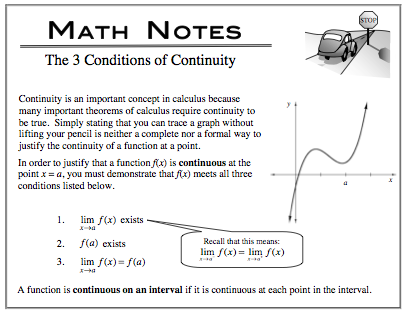### Home > APCALC > Chapter 5 > Lesson 5.2.5 > Problem5-104

5-104.

Let $f\left(x\right)=x^{ 1/3}$. Homework Help ✎

1. Use the formal definition of continuity to determine if f is continuous at x = 0.2. Calculate $\lim\limits _ { x \rightarrow 0 } \frac { f ( x ) - f ( 0 ) } { x - 0 }$.

This is Ana's definition of the derivative. The limit is equivalent to f '(0).So you can use the power rule to find the limit, if it exists.

3. What does the limit in part (b) represent?

Refer to the hint in part (b).

4. What is happening graphically at x = 0 that causes your answer to part (b)?

Recall that derivatives do not exist at locations where f(x) has a: cusp, endpoint, jump, hole or vertical tangent.

Sketching the graph may help visualize the issue.